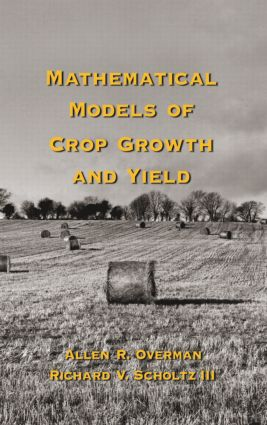Mathematical Models of Crop Growth and Yield

1st Edition

CRC Press

344 pages

Hardback: 9780824708252
pub: 2002-08-27
\$145.00
x
eBook (VitalSource) : 9780429221705
pub: 2002-08-27
from \$72.50

FREE Standard Shipping!

Description

Highlighting effective, analytical functions that have been found useful for the comparison of alternative management techniques to maximize water and nutrient resources, this reference describes the application of viable mathematical models in data analysis to increase crop growth and yields. Featuring solutions to various differential equations, the book covers the characteristics of the functions related to the phenomenological growth model. Including more than 1300 literature citations, display equations, tables, and figures and outlining an approach to mathematical crop modeling, Mathematical Models of Crop Growth and Yield will prove an invaluable resource.

INTRODUCTION

Historic Background

Yield Response Models

Growth Models

Environmental Input

Summary

Exercises

References

SEASONAL RESPONSE MODELS

Background

Extended Logistic Model

Extended Multiple Logistic Model

Water Availability

Legume/Grass Interaction

Summary

Exercises

References

GROWTH RESPONSE MODELS

Background

Empirical Growth Model

Extended Empirical Growth Model

Phenomenological Growth Model

Expanded Growth Model

Exercises

References

MATHEMATICAL CHARACTERISTICS OF MODELS

Background

Phenomenological Growth Model

Expanded Growth Model

Rational Basis for Logistic Model

Coupling Among Applied Soil and Plant Components

Accumulation of Dry Matter and Plant Nutrients

Exercises

References

PASTURE SYSTEMS

Background

Linear Exponential Model

Summary

Exercises

References

NONLINEAR REGRESSION FOR MATHEMATICAL MODELS

Background

Logistic Model

Probability Model

Confidence Contours

Sensitivity Analysis

Dimensionless Plots

Correlation Coefficient

Exercises

References

Index